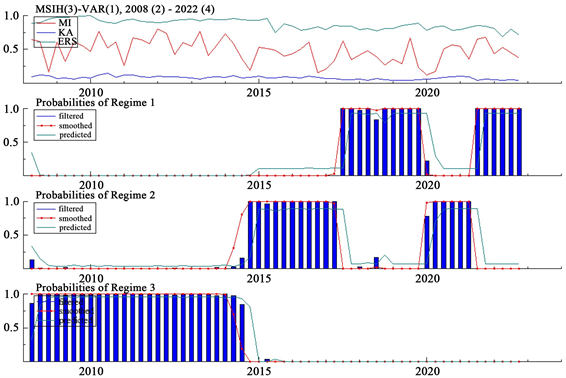#### 期刊菜单

The “Non-Corner Solution” of the Trilemma and China’s Macroeconomic Policy Portfolio—An Empirical Analysis Based on the MS-VAR Model
DOI: 10.12677/FIN.2023.135123, PDF , HTML, XML, 下载: 93  浏览: 151

Abstract: With the continuous development of the Chinese economy, the People’s Bank of China has adopted different macroeconomic policy portfolios to cooperate. As a classic analytical framework of macro policy, the traditional Trilemma does not specify the primary and secondary as well as the development trend of the ternary policy. By constructing an MS-VAR model, we can delineate the actual configuration pattern of China’s Trilemma and utilize it to study the macroeconomic policy mix under different regimes in China. According to the regression results, exchange rate stability emerges as the primary determinant of China’s Trilemma policy, followed by the level of capital account openness, and then monetary policy independence. Therefore, when choosing the policy portfolio, the People’s Bank of China can gradually promote the free flow of capital on the basis of maintaining the stability of the exchange rate, and allocate the weight of the Trilemma policy objectives according to China’s actual situation, so as to promote the sound development of China’s economy.

1. 引言

2. 三元悖论理论的修正

 (1)

3. 基于MS-VAR模型的我国三元悖论实际配置格局划分

3.1. 三元悖论中三个政策指标的测度

1) 货币政策独立性

$corr\left({r}_{i},{r}_{b}\right)$ 表示中国与中心国(美国)的利率相关系数。MI取值介于0和1之间，MI取值越小代表本国和中心国的基准利率的相关性越强，从而本国的货币政策独立性越弱，反之就越强。

2) 汇率稳定性

$exch\text{_}rate$ 表示直接标价法下中国货币与基准货币(美元)之间的名义汇率，stdev(.)表示关于标准差的函数。ERS取值介于0和1之间，ERS取值越大代表本国的汇率稳定性越强，反之越弱。

3) 资本账户开放程度

Lane PR & Milesi-Ferretti GM (2007)  提出用外国直接投资和证券投资的资产和负债总量占GDP的比重来衡量资本账户开放程度。本文基于中国实际情况的考虑，在其基础上对资本账户开放程度进行测度，即金融直接投资账户(DI)、证券投资账户(SI)、衍生工具账户(FI)、其他投资账户(OI)四项之和占GDP的比值来表示中国资本账户开放水平。对该指标表述如下： $\text{KA}=\left(\text{DI}+\text{SI}+\text{FI}+\text{OI}\right)/\text{GDP}$

KA取值介于0和1之间，KA取值越大代表本国的资本流动程度越大，反之越小。

3.2. 三元悖论三元政策区制状态分析

MS-VAR模型的一般形式可以表述为： ${y}_{t}-\mu \left({s}_{t}\right)={A}_{1}\left({s}_{t}\right)\left({y}_{t-1}-\mu \left({s}_{t-1}\right)\right)+\cdots +{A}_{p}\left({s}_{t}\right)\left({y}_{t-p}-\mu \left({s}_{t-p}\right)\right)+{u}_{t}$ 其中， ${u}_{t}I{s}_{t}\sim NID\left(0,\sum \left({s}_{t}\right)\right)$ ，参数转移函数 $\mu \left({s}_{t}\right),\sum \left({s}_{t}\right),{A}_{1}\left({s}_{t}\right),\cdots ,{A}_{p}\left({s}_{t}\right)$ 依赖于区制变量 ${s}_{t}$ 所处的状态： $\mu \left({s}_{t}\right)=\left\{\left({\mu }_{1},{s}_{t}=1\right),\cdots ,\left({\mu }_{p},{s}_{t}=M\right)\right\}$ 其中，区制变量 ${s}_{t}\in \left\{1,\cdots ,M\right\}$ 服从离散时间、离散状态的马尔可夫链过程，各状态间的转变通过转移概率表示，从区制i到区制j的转移概率为： ${p}_{ij}={P}_{r}\left({s}_{t+1}=jI{s}_{t}=i\right),\text{}{\sum }_{j=1}^{M}{p}_{ij}=1,\text{}\forall i,j\in \left\{1,\cdots ,M\right\}$

MS-VAR模型要求所有变量都为平稳时间序列，因此需考察货币政策独立性(MI)、汇率稳定性(ERS)、资本账户开放程度(KA)的平稳性。从表1中可以看出，资本流动程度指标KA、汇率稳定性指标ERS和货币政策独立性指标MI均在1%的显著性水平上平稳。故本文研究涉及的三个研究变量(KA, ERS, MI)均平稳，不会出现伪相关与伪回归问题。Table 1. Results of a stationary test for trilemma variablesTable 2. MS-VAR model selection basisFigure 1. MSIH-VAR model zone system conversion filter probability, smoothing probability, and prediction probability plotsTable 3. MSIA-VAR model zone conversion propertiesTable 4. Zonal transfer probability matrix for MSIA-VAR model

4. 不同区制状态下的我国宏观经济政策组合Table 5. Zonal linear constraint regression results

Standard errors in parentheses；***p < 0.01，**p < 0.05，*p < 0.1。

5. 结语

  保罗·克鲁格曼, 朱文晖, 王玉清. 萧条经济学的回归[M]. 北京: 中国人民大学出版社, 1999.  易纲, 汤弦. 汇率制度的“角点解假设”的一个理论基础[J]. 金融研究, 2001(8): 5-17.  Rey, H. (2015) Dilemma Not Trilem-ma: The Global Financial Cycle and Monetary Policy Independence. National Bureau of Economic Research, No. w21162. https://doi.org/10.3386/w21162  Aizenman, J. (2010) The Impossible Trinity (aka the Policy Trilem-ma): The Encyclopedia of Financial Globalization. Economics Department, University of California, Working Paper, No. 666. http://hdl.handle.net/10419/64487  Steiner, A. (2017) Central Banks and Macroeconomic Policy Choices: Re-laxing the Trilemma. Journal of Banking & Finance, 77, 283-299. https://doi.org/10.1016/j.jbankfin.2015.07.005  周姝彤. 汇率制度选择的国际经验——基于三元悖论分析[J]. 工业技术经济, 2022, 41(3): 98-105.  杨荣海, 石健. “三元悖论”视角下人民币加入SDR后的金融安全分析[J]. 经济学家, 2020(9): 32-41.  管涛. “三元悖论”的中国解决方案: 稳汇率与稳增长的现实选择[J]. 清华金融评论, 2019(4): 33-35.  Aizenman, J., Chinn, M.D. and Ito, H. (2008) Assessing the Emerging Global Finan-cial Architecture: Measuring the Trilemma’s Configurations over Time. National Bureau of Economic Research, No. w14533. https://doi.org/10.3386/w14533  Lane, P.R. and Milesi-Ferretti, G.M. (2007) The External Wealth of Nations Mark II: Revised and Extended Estimates of Foreign Assets and Liabilities, 1970-2004. Journal of international Eco-nomics, 73, 223-250. https://doi.org/10.1016/j.jinteco.2007.02.003